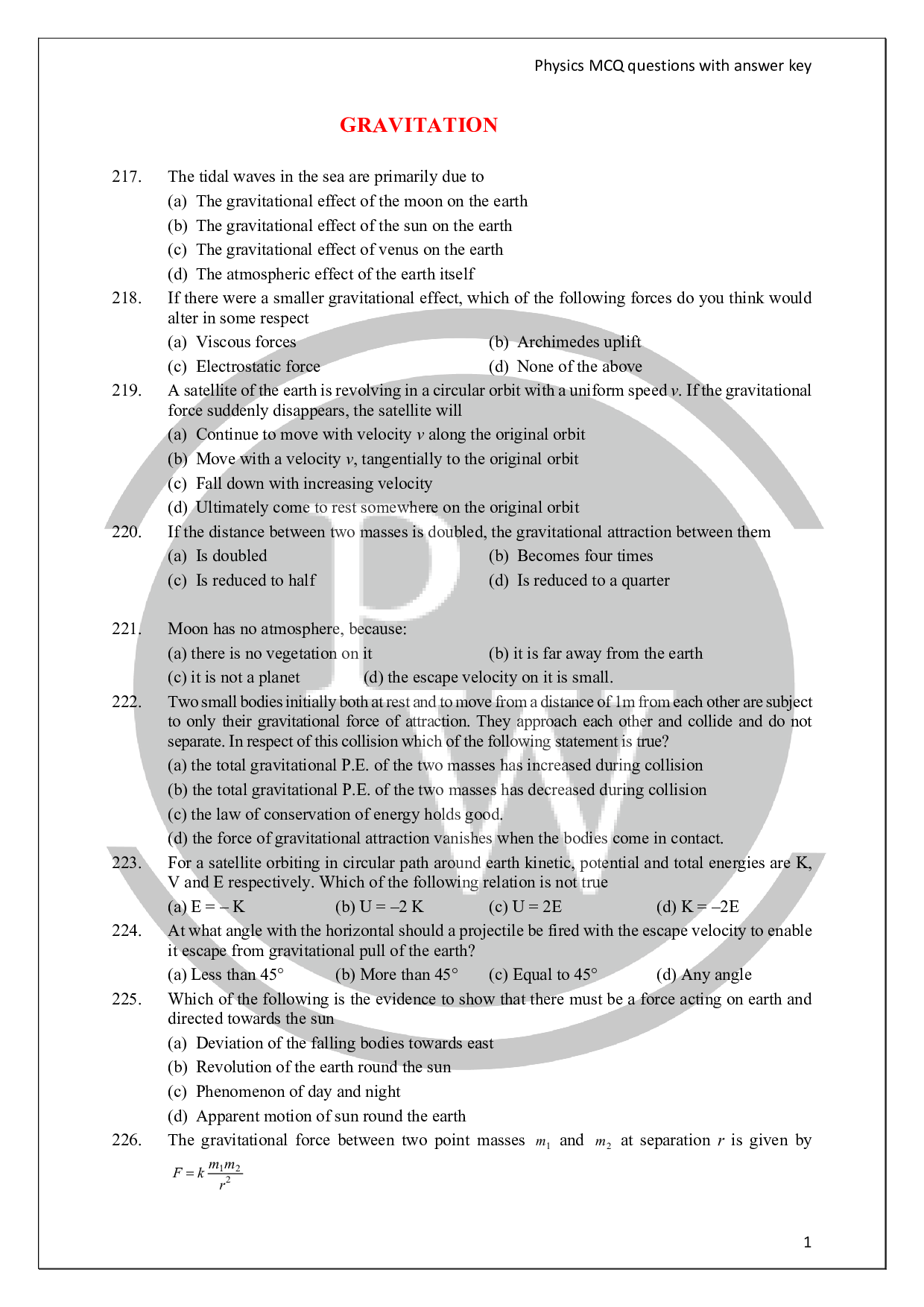# Gravitation questions

So far we have discussed various forces: Pushes and pulls, elastic forces, friction and other forces that act when one body is in contact with another. In this lesson we study the properties of one particularly important non-contact force, gravitation which is one of the fundamental and universal force of nature. The law that describes the gravitational forces between any two bodies was discovered by Newton. As you go through this lesson, you will note many of the basic concepts of dynamics, discussed in various lessons. In particular, we shall use Newton’s force laws, dynamics of circular motion, potential energy, conservation of energy & angular momentum.Do check out chapter wise Physics Questions prepared by Entrancei.

### Find below Gravitation questions pdf